# Algebraic Expressions solutions class 7 chapter 10 exercise 10.2 NCERT maths

NCERT Mathematics class 7th chapter 10 Algebraic Expressions Exercise 10.2 solutions are given.

You should study the textbook lesson Algebraic Expressions very well.

You should also observe and practice all example problems and solutions are given in the textbook

Observe the given below solutions and try them in your own method.

Algebraic Expressions

## Class 7 maths exercise 10.2 solutions NCERT

Algebraic Expressions

Chapter 10

Exercise 10.2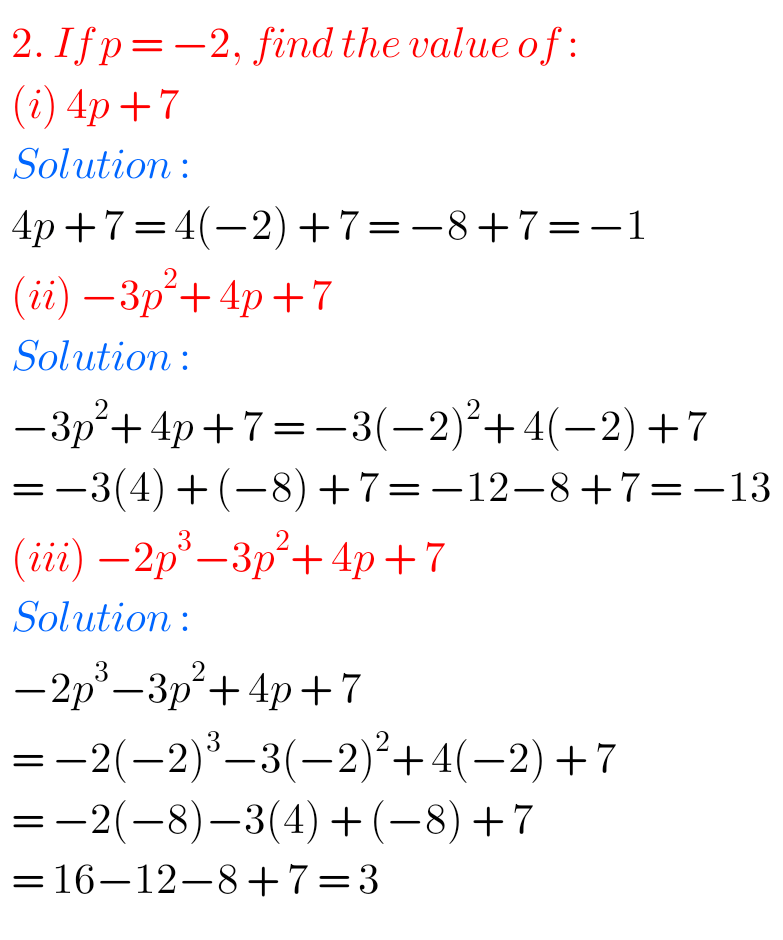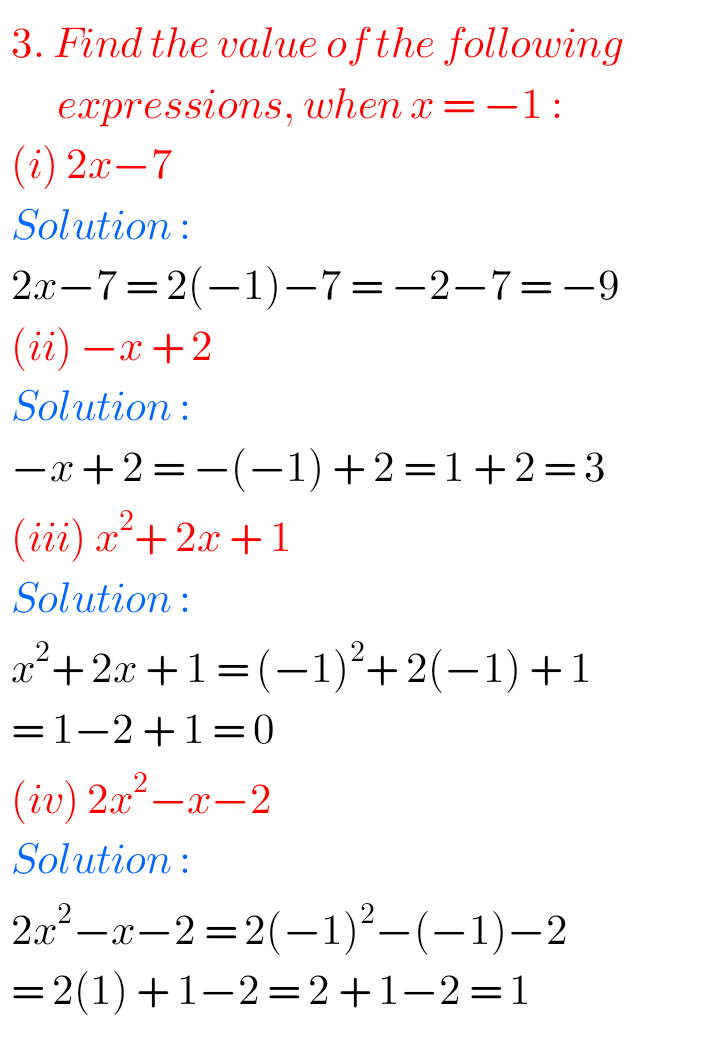### Solutions for Algebraic Expressions class 7 maths NCERT Exercise 10.2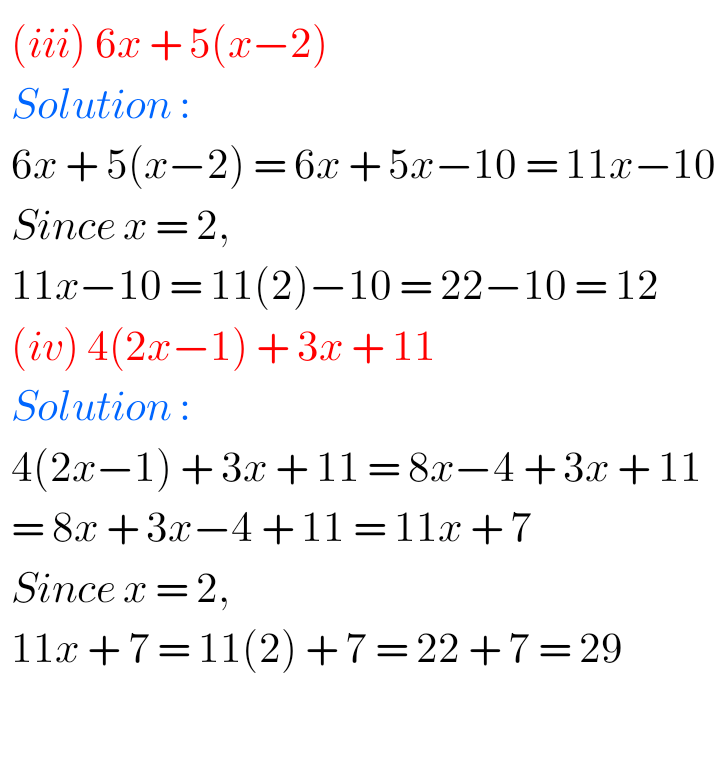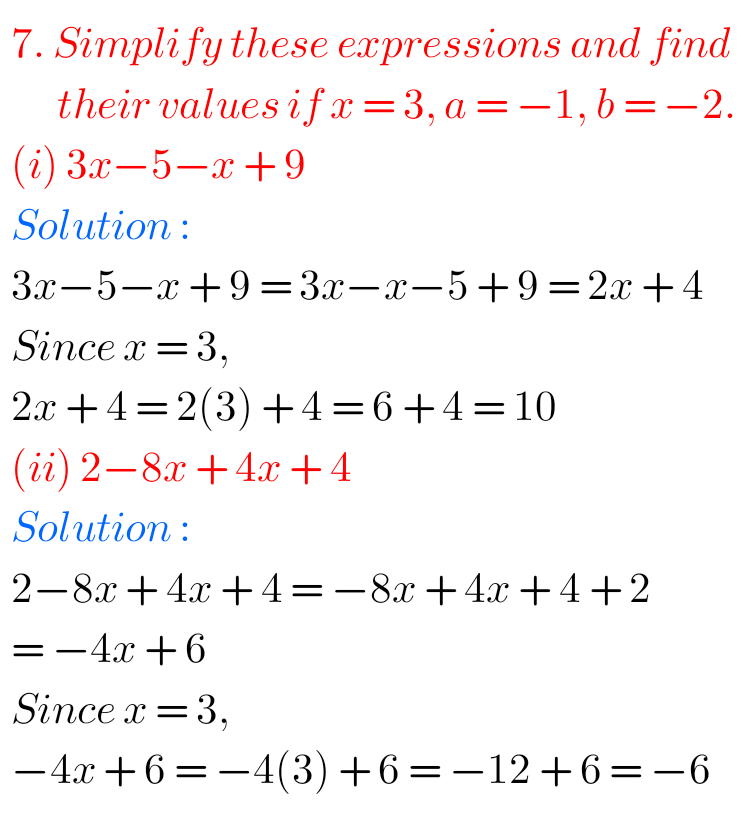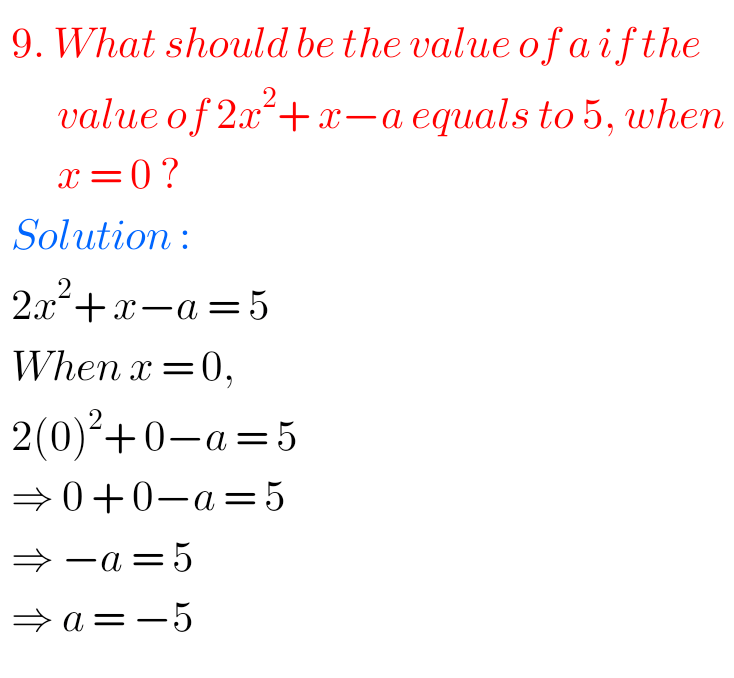Note : Observe the solutions and try them in your own method.

Inter Maths solutions

SSC Maths Class 10 solutions

NCERT class 6 maths solutions